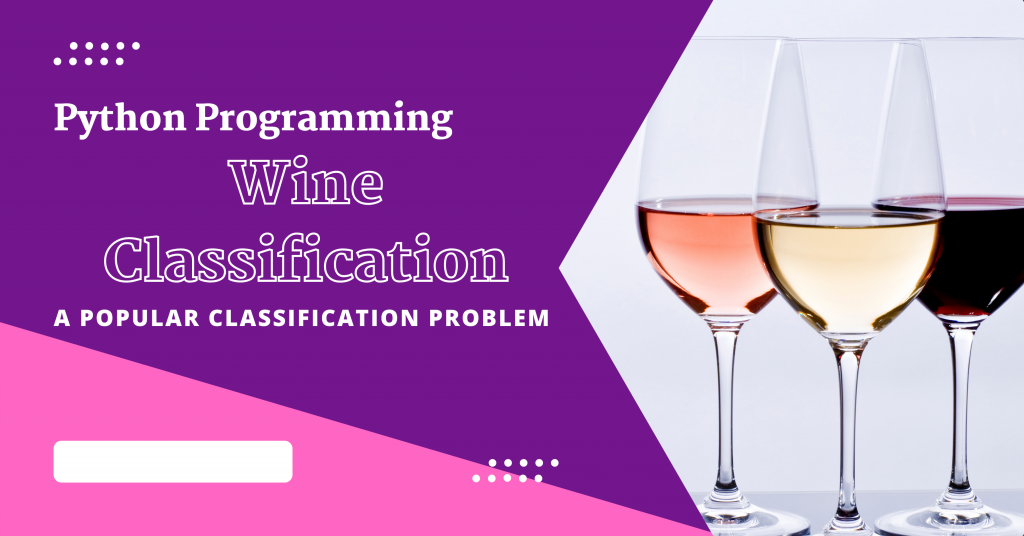# Wine Classification using Python – Easily ExplainedHello everybody! In this tutorial, we are going to learn how to classify wines on the basis of various features in the Python programming language.

Also read: Classifying Clothing Images in Python – A complete guide

## Introduction to Wine Classification

There are numerous wines available in this globe, including dessert wines, sparkling wines, appetizers, pop wines, table wines, and vintage wines.

You may wonder how one knows which wine is good and which is not. The answer to this question is machine learning!

There are numerous wine categorization methods available. Here are listed a few of them:

1. CART
2. Logistic Regression
3. Random forest
4. Naïve Bayes
5. Perception
6. SVM
7. KNN

## Implementing Wine Classification in Python

Let’s now get into a very basic implementation of a wine classifier in Python. This will give you a starting point in learning how classifiers work and how you can implement them in Python for various real-world scenarios.

### 1. Importing Modules

The first step is importing all the necessary modules/libraries into the program. The modules needed for the classification are some basic modules such as:

The next step is to import all the models into the program that comes under the sklearn library. We will also include some other functions from the sklearn library.

The models loaded are listed below:

1. SVM
2. Logistic Regression
```import numpy as np
import pandas as pd
import matplotlib.pyplot as plt
from sklearn import svm
from sklearn import metrics
from sklearn.linear_model import LogisticRegression
from sklearn.metrics import classification_report
from sklearn.metrics import confusion_matrix,accuracy_score
from sklearn.preprocessing import normalize
```

### 2. Dataset Preparation

Next, we need to prepare our dataset. Let me begin by introducing the dataset and then importing the same in our application.

#### 2.1 Introduction to Dataset

In the dataset, we have 6497 observations and in total 12 features. There aren’t NAN values in any variable. You can download the data easily here.

The name and description of the 12 features are as follows:

• Fixed acidity: Amount of acidity in the wine
• Volatile acidity: Amount of acetic acid present in the wine
• Citric acid: Amount of citric acid present in the wine
• Residual sugar: Amount of sugar after fermentation
• Chlorides: Amount of salts present in the wine
• Free sulfur dioxide: Amount of free form of SO2
• Total sulfur dioxide: Amount of free and bound forms of S02
• Density: Density of the wine (mass/volume)
• pH: pH of the wine ranging from 0-14
• Sulphates: Amount of sulfur dioxide gas (S02) levels in the wine
• Alcohol: Amount of alcohol present in the wine
• Quality: Final quality of the wine mentioned

Dataset is loaded into the program with the help of the `read_csv` function and display the first five rows of the dataset using the `head` function.

```data=pd.read_csv("./wine_dataset.csv")
```

#### 2.3 Cleaning of Data

Cleaning of the dataset includes dropping the unnecessary columns and the NaN values with the help of the code mentioned below:

```data=data.drop('Unnamed: 0',axis=1)
data.dropna()
```

#### 2.4 Data Visualization

An important step is to first visualize the data before processing it any further. The visualization is done in two forms namely,

1. Histographs
2. Seaborn Graph
##### Plotting Histograms
```plt.style.use('dark_background')
colors=['blue','green','red','cyan','magenta','yellow','blue','green','red','magenta','cyan','yellow']
plt.figure(figsize=(20,50))
for i in range(1,13):
plt.subplot(6,6,i)
plt.hist(data[data.columns[i-1]],color=colors[i-1])
plt.xlabel(data.columns[i-1])
plt.show()
```

We will be plotting histograms for each feature separately. The output is displayed below.

##### Plotting Seaborn
```import seaborn as sns
plt.figure(figsize=(10,10))
correlations = data[data.columns].corr(method='pearson')
sns.heatmap(correlations, annot = True)
plt.show()
```

Seaborn graphs are used to show the relationship between different features present in the dataset.

#### 2.5 Train-Test Split and Data Normalization

To split the data into training and testing data, there is no optimal splitting percentage.

But one of the fair splitting rules is the 80/20 rule where 80% of the data goes to training data and the rest 20% goes to testing data.

This step also involves normalizing the dataset.

```split=int(0.8*data.shape)
print("Split of data is at: ",split)
print("\n-------AFTER SPLITTING-------")
train_data=data[:split]
test_data=data[split:]
print('Shape of train data:',train_data.shape)
print('Shape of train data:',test_data.shape)
print("\n----CREATING X AND Y TRAINING TESTING DATA----")
y_train=train_data['quality']
y_test=test_data['quality']
x_train=train_data.drop('quality',axis=1)
x_test=test_data.drop('quality',axis=1)
print('Shape of x train data:',x_train.shape)
print('Shape of y train data:',y_train.shape)
print('Shape of x test data:',x_test.shape)
print('Shape of y test data:',y_test.shape)

nor_train=normalize(x_train)
nor_test=normalize(x_test)
```

### 3. Wine Classification Model

In this program we have used two algorithms namely, SVM and Logistic Regression.

#### 3.1 Support Vector Machine (SVM) Algorithm

```clf = svm.SVC(kernel='linear')
clf.fit(nor_train, y_train)
y_pred_svm = clf.predict(nor_test)
print("Accuracy (SVM) :",metrics.accuracy_score(y_test, y_pred_svm)*100)
```

The accuracy of the model turned out to be around `50%`.

#### 3.2 Logistic Regression Algorithm

```logmodel = LogisticRegression()
logmodel.fit(nor_train, y_train)
y_pred_LR= logmodel.predict(nor_test)
print('Mean Absolute Error(Logistic Regression):', metrics.mean_absolute_error(y_test, y_pred_LR)*100)
```

The accuracy, in this case, turns out to be around `50%` as well. The main reason for this is the model that we’ve used/created. Advanced models such as those available for tensorflow are

## Conclusion

In order to get higher accuracy, you can check out tensorflow models as well!

Happy Learning! 😇

Stay tuned for more such tutorials! Thank you for reading!